Previous Page | Next Page

 The GLIMMIX Procedure

## Example 38.4 Quasi-likelihood Estimation for Proportions with Unknown Distribution

Wedderburn (1974) analyzes data on the incidence of leaf blotch (Rhynchosporium secalis) on barley. The data represent the percentage of leaf area affected in a two-way layout with 10 barley varieties at nine sites. The following DATA step converts these data to proportions, as analyzed in McCullagh and Nelder (1989, Ch. 9.2.4). The purpose of the analysis is to make comparisons among the varieties, adjusted for site effects.

```data blotch;
array p{9} pct1-pct9;
input variety pct1-pct9;
do site = 1 to 9;
prop = p{site}/100;
output;
end;
drop pct1-pct9;
datalines;
1  0.05  0.00  1.25  2.50  5.50  1.00  5.00  5.00 17.50
2  0.00  0.05  1.25  0.50  1.00  5.00  0.10 10.00 25.00
3  0.00  0.05  2.50  0.01  6.00  5.00  5.00  5.00 42.50
4  0.10  0.30 16.60  3.00  1.10  5.00  5.00  5.00 50.00
5  0.25  0.75  2.50  2.50  2.50  5.00 50.00 25.00 37.50
6  0.05  0.30  2.50  0.01  8.00  5.00 10.00 75.00 95.00
7  0.50  3.00  0.00 25.00 16.50 10.00 50.00 50.00 62.50
8  1.30  7.50 20.00 55.00 29.50  5.00 25.00 75.00 95.00
9  1.50  1.00 37.50  5.00 20.00 50.00 50.00 75.00 95.00
10 1.50 12.70 26.25 40.00 43.50 75.00 75.00 75.00 95.00
;
```

Little is known about the distribution of the leaf area proportions. The outcomes are not binomial proportions, because they do not represent the ratio of a count over a total number of Bernoulli trials. However, because the mean proportion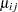for varietyon sitemust lie in the interval
, you can commence the analysis with a model that treats Prop as a "pseudo-binomial" variable: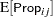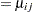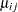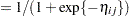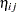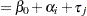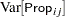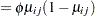Here,is the linear predictor for varietyon site,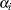denotes theth site effect, and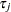denotes theth barley variety effect. The logit of the expected leaf area proportions is linearly related to these effects. The variance funcion of the model is that of a binomial(,) variable, and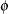is an overdispersion parameter. The moniker "pseudo-binomial" derives not from the pseudo-likelihood methods used to estimate the parameters in the model, but from treating the response variable as if it had first and second moment properties akin to a binomial random variable.

The model is fit in the GLIMMIX procedure with the following statements:

```proc glimmix data=blotch;
class site variety;
model prop = site variety / link=logit dist=binomial;
random _residual_;
lsmeans variety / diff=control('1');
run;
```

The MODEL statement specifies the distribution as binomial and the logit link. Because the variance function of the binomial distribution is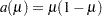, you use the statement

```
random _residual_;
```

to specify the scale parameter. The LSMEANS statement requests estimates of the least squares means for the barley variety. The DIFF=CONTROL(’1’) option requests tests of least squares means differences against the first variety.

The "Model Information" table in Output 38.4.1 describes the model and methods used in fitting the statistical model. It is assumed here that the data are binomial proportions.

Output 38.4.1 Model Information in Pseudo-binomial Analysis
The GLIMMIX Procedure

Model Information
Data Set WORK.BLOTCH
Response Variable prop
Response Distribution Binomial
Variance Function Default
Variance Matrix Diagonal
Estimation Technique Maximum Likelihood
Degrees of Freedom Method Residual

The "Class Level Information" table in Output 38.4.2 lists the number of levels of the Site and Variety effects and their values. All 90 observations read from the data are used in the analysis.

Output 38.4.2 Class Levels and Number of Observations
Class Level Information
Class Levels Values
site 9 1 2 3 4 5 6 7 8 9
variety 10 1 2 3 4 5 6 7 8 9 10

 Number of Observations Read 90 90

In Output 38.4.3, the "Dimensions" table shows that the model does not contain G-side random effects. There is a single covariance parameter, which corresponds to. The "Optimization Information" table shows that the optimization comprises 18 parameters (Output 38.4.3). These correspond to the 18 nonsingular columns of the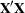matrix.

Output 38.4.3 Model Fit in Pseudo-binomial Analysis
Dimensions
Covariance Parameters 1
Columns in X 20
Columns in Z 0
Subjects (Blocks in V) 1
Max Obs per Subject 90

Optimization Information
Optimization Technique Newton-Raphson
Parameters in Optimization 18
Lower Boundaries 0
Upper Boundaries 0
Fixed Effects Not Profiled

Fit Statistics
-2 Log Likelihood 57.15
AIC (smaller is better) 93.15
AICC (smaller is better) 102.79
BIC (smaller is better) 138.15
CAIC (smaller is better) 156.15
HQIC (smaller is better) 111.30
Pearson Chi-Square 6.39
Pearson Chi-Square / DF 0.09

There are significant site and variety effects in this model based on the approximate Type IIItests (Output 38.4.4).

Output 38.4.4 Tests of Site and Variety Effects in Pseudo-binomial Analysis
Type III Tests of Fixed Effects
Effect Num DF Den DF F Value Pr > F
site 8 72 18.25 <.0001
variety 9 72 13.85 <.0001

Output 38.4.5 displays the Variety least squares means for this analysis. These are obtained by averaging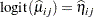across the sites. In other words, LS-means are computed on the linked scale where the model effects are additive. Note that the least squares means are ordered by variety. The estimate of the expected proportion of infected leaf area for the first variety is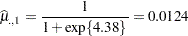and that for the last variety is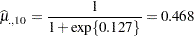Output 38.4.5 Variety Least Squares Means in Pseudo-binomial Analysis
variety Least Squares Means
variety Estimate Standard Error DF t Value Pr > |t|
1 -4.3800 0.5643 72 -7.76 <.0001
2 -4.2300 0.5383 72 -7.86 <.0001
3 -3.6906 0.4623 72 -7.98 <.0001
4 -3.3319 0.4239 72 -7.86 <.0001
5 -2.7653 0.3768 72 -7.34 <.0001
6 -2.0089 0.3320 72 -6.05 <.0001
7 -1.8095 0.3228 72 -5.61 <.0001
8 -1.0380 0.2960 72 -3.51 0.0008
9 -0.8800 0.2921 72 -3.01 0.0036
10 -0.1270 0.2808 72 -0.45 0.6523

Because of the ordering of the least squares means, the differences against the first variety are also ordered from smallest to largest (Output 38.4.6).

Output 38.4.6 Variety Differences against the First Variety
Differences of variety Least Squares Means
variety _variety Estimate Standard Error DF t Value Pr > |t|
2 1 0.1501 0.7237 72 0.21 0.8363
3 1 0.6895 0.6724 72 1.03 0.3086
4 1 1.0482 0.6494 72 1.61 0.1109
5 1 1.6147 0.6257 72 2.58 0.0119
6 1 2.3712 0.6090 72 3.89 0.0002
7 1 2.5705 0.6065 72 4.24 <.0001
8 1 3.3420 0.6015 72 5.56 <.0001
9 1 3.5000 0.6013 72 5.82 <.0001
10 1 4.2530 0.6042 72 7.04 <.0001

This analysis depends on your choice for the variance function that was implied by the binomial distribution. You can diagnose the distributional assumption by examining various graphical diagnotics measures. The following statements request a panel display of the Pearson-type residuals:

```ods graphics on;
ods select PearsonPanel;
proc glimmix data=blotch plots=pearsonpanel;
class site variety;
model prop = site variety / link=logit dist=binomial;
random _residual_;
run;
ods graphics off;
```

Output 38.4.7 clearly indicates that the chosen variance function is not appropriate for these data. Asapproaches zero or one, the variability in the residuals is less than that implied by the binomial variance function.

Output 38.4.7 Panel of Pearson-Type Residuals in Pseudo-binomial Analysis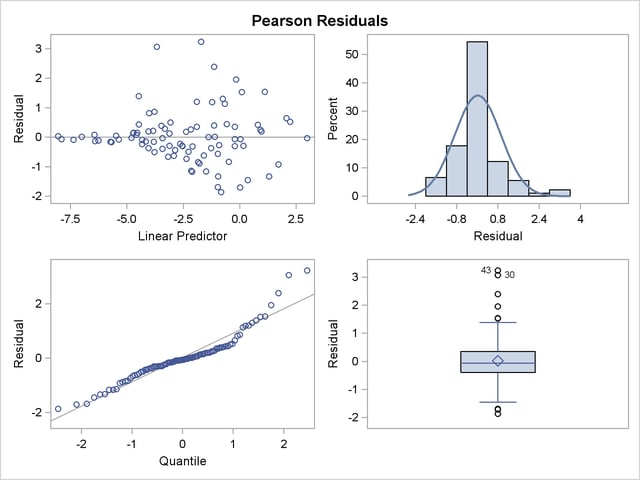To remedy this situation, McCullagh and Nelder (1989) consider instead the variance function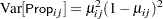Imagine two varieties with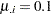and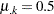. Under the binomial variance function, the variance of the proportion for varietyis 2.77 times larger than that for variety. Under the revised model this ratio increases to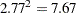.

The analysis of the revised model is obtained with the next set of GLIMMIX statements. Because you need to model a variance function that does not correspond to any of the built-in distributions, you need to supply a function with an assignment to the automatic variable _VARIANCE_. The GLIMMIX procedure then considers the distribution of the data as unknown. The corresponding estimation technique is quasi-likelihood. Because this model does not include an extra scale parameter, you can drop the RANDOM _RESIDUAL_ statement from the analysis.

```ods graphics on;
ods select ModelInfo FitStatistics LSMeans Diffs PearsonPanel;
proc glimmix data=blotch plots=pearsonpanel;
class site variety;
_variance_ = _mu_**2 * (1-_mu_)**2;
model prop = site variety / link=logit;
lsmeans variety / diff=control('1');
run;
ods graphics off;
```

The "Model Information" table in Output 38.4.8 now displays the distribution as "Unknown," because of the assignment made in the GLIMMIX statements to _VARIANCE_. The table also shows the expression evaluated as the variance function.

Output 38.4.8 Model Information in Quasi-likelihood Analysis
The GLIMMIX Procedure

Model Information
Data Set WORK.BLOTCH
Response Variable prop
Response Distribution Unknown
Variance Function _mu_**2 * (1-_mu_)**2
Variance Matrix Diagonal
Estimation Technique Quasi-Likelihood
Degrees of Freedom Method Residual

The fit statistics of the model are now expressed in terms of the log quasi-likelihood. It is computed as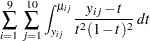Twice the negative of this sum equals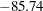, which is displayed in the "Fit Statistics" table (Output 38.4.9).

The scaled Pearson statistic is now 0.99. Inclusion of an extra scale parameterwould have little or no effect on the results.

Output 38.4.9 Fit Statistics in Quasi-likelihood Analysis
Fit Statistics
-2 Log Quasi-Likelihood -85.74
Quasi-AIC (smaller is better) -49.74
Quasi-AICC (smaller is better) -40.11
Quasi-BIC (smaller is better) -4.75
Quasi-CAIC (smaller is better) 13.25
Quasi-HQIC (smaller is better) -31.60
Pearson Chi-Square 71.17
Pearson Chi-Square / DF 0.99

The panel of Pearson-type residuals now shows a much more adequate distribution for the residuals and a reduction in the number of outlying residuals (Output 38.4.10).

Output 38.4.10 Panel of Pearson-Type Residuals (Quasi-likelihood)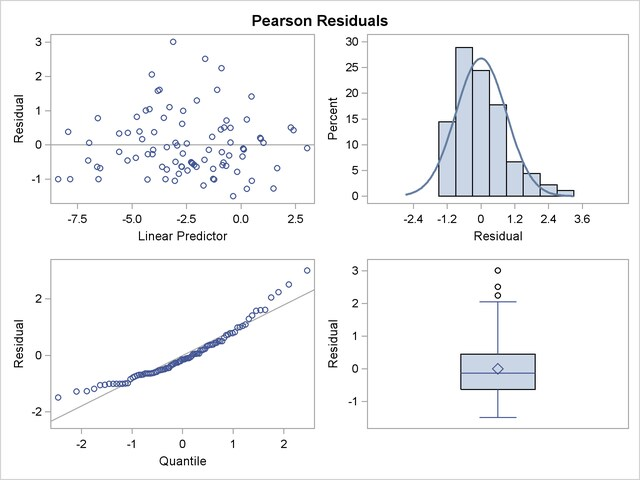The least squares means are no longer ordered in size by variety (Output 38.4.11). For example,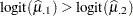. Under the revised model, the second variety has a greater percentage of its leaf area covered by blotch, compared to the first variety. Varieties 5 and 6 and varieties 8 and 9 show similar reversal in ranking.

Output 38.4.11 Variety Least Squares Means in Quasi-likelihood Analysis
variety Least Squares Means
variety Estimate Standard Error DF t Value Pr > |t|
1 -4.0453 0.3333 72 -12.14 <.0001
2 -4.5126 0.3333 72 -13.54 <.0001
3 -3.9664 0.3333 72 -11.90 <.0001
4 -3.0912 0.3333 72 -9.27 <.0001
5 -2.6927 0.3333 72 -8.08 <.0001
6 -2.7167 0.3333 72 -8.15 <.0001
7 -1.7052 0.3333 72 -5.12 <.0001
8 -0.7827 0.3333 72 -2.35 0.0216
9 -0.9098 0.3333 72 -2.73 0.0080
10 -0.1580 0.3333 72 -0.47 0.6369

Interestingly, the standard errors are constant among the LS-means (Output 38.4.11) and among the LS-means differences (Output 38.4.12). This is due to the fact that for the logit link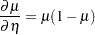which cancels with the square root of the variance function in the estimating equations. The analysis is thus orthogonal.

Output 38.4.12 Variety Differences in Quasi-likelihood Analysis
Differences of variety Least Squares Means
variety _variety Estimate Standard Error DF t Value Pr > |t|
2 1 -0.4673 0.4714 72 -0.99 0.3249
3 1 0.07885 0.4714 72 0.17 0.8676
4 1 0.9541 0.4714 72 2.02 0.0467
5 1 1.3526 0.4714 72 2.87 0.0054
6 1 1.3286 0.4714 72 2.82 0.0062
7 1 2.3401 0.4714 72 4.96 <.0001
8 1 3.2626 0.4714 72 6.92 <.0001
9 1 3.1355 0.4714 72 6.65 <.0001
10 1 3.8873 0.4714 72 8.25 <.0001Previous Page | Next Page | Top of Page